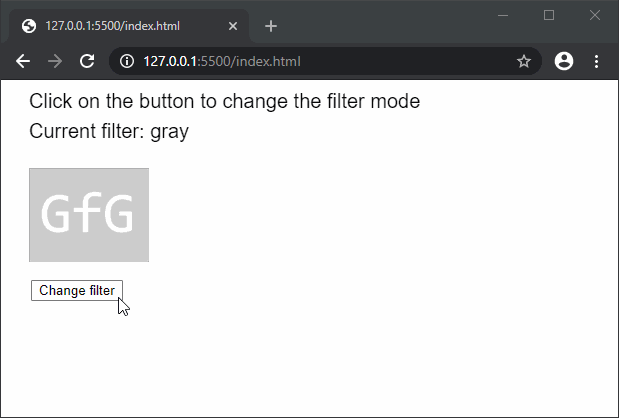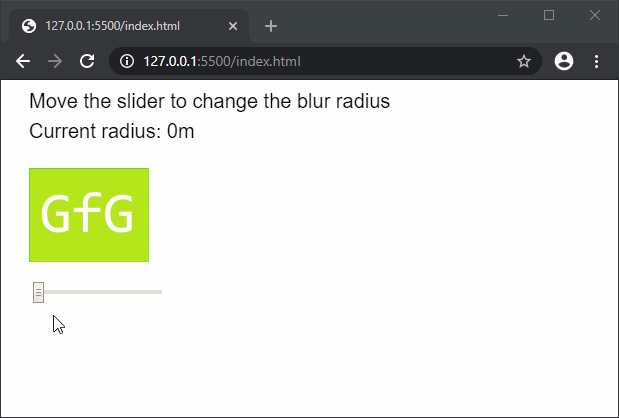Open in App
Not now

# p5.js | filter() Function

• Last Updated : 27 Apr, 2021

The filter() function is used to apply filters to the canvas. p5.js has several presets that can be used with different levels of intensity to get the desired effect.
Syntax:

`filter( filterType, filterParam)`

Parameters: This function accept two parameters as mentioned above and described below:

• filterType: It is constant which defines the preset to be used as the filter. It can have the following values:
• THRESHOLD: This mode is used to convert the image to black and white depending on the threshold defined in the optional level parameter. A level value of 0.0 means that the image would be completely black and a value of 1.0 means that the image would be white. In case no value is specified, 0.5 is used.
• GRAY: This mode converts all colors in the image to grayscale equivalents. The optional parameter is not used for this preset.
• OPAQUE: This mode sets the alpha channel of the image to be entirely opaque. The optional parameter is not used for this preset.
• INVERT: This mode inverts each of the pixels in the image. The optional parameter is not used for this preset.
• POSTERIZE: This mode limits each channel of the image to the number of colors as specified in the value. The optional parameter can be set in the range of 2 to 255, with the most noticeable effects at the lower color ranges.
• BLUR: This mode applies a gaussian blur to the image. The optional parameter is used to specify the strength of the blur. If no parameter is specified, then a gaussian blur with a radius of 1 metre is applied.
• ERODE: This mode reduces the light areas. The optional parameter is not used for this preset.
• DILATE: This mode increases the light areas. The optional parameter is not used for this preset.
• filterParam: It is a number which is unique to each filter and affects the functionality of the filter. It is an optional parameter.

Below examples illustrate the filter() function in p5.js:
Example 1:

## javascript

 `function` `preload() {` `  ``img = loadImage(``"sample-image.png"``);` `}`   `function` `setup() {` `  ``filterModes = [` `    ``GRAY,` `    ``OPAQUE,` `    ``INVERT,` `    ``POSTERIZE,` `    ``BLUR,` `    ``ERODE,` `    ``DILATE,` `    ``BLUR,` `    ``THRESHOLD` `  ``];`   `  ``index = 0;` `  ``currFilterMode = filterModes[index];`   `  ``createCanvas(500, 300);` `  ``textSize(20);`   `  ``btn = createButton(``"Change filter"``);` `  ``btn.position(30, 200);` `  ``btn.mousePressed(changeFilter);` `}`   `function` `draw() {` `  ``clear();`   `  ``text(``'Click on the button to change the filter mode'``, 20, 20);` `  ``text(``"Current filter: "` `+ currFilterMode, 20, 50);`   `  ``image(img, 20, 80);`   `  ``// Set the filter` `  ``filter(currFilterMode);` `}`   `function` `changeFilter() {` `  ``if` `(index < filterModes.length - 1)` `    ``index++;` `  ``else` `    ``index = 0;` `  ``currFilterMode = filterModes[index];`   `  ``console.log(``"Current filter: "` `+ currFilterMode);` `}`

Output:Example 2:

## javascript

 `let level = 0;`   `function` `preload() {` `  ``img = loadImage(``"sample-image.png"``);` `}`   `function` `setup() {` `  ``createCanvas(500, 300);` `  ``textSize(20);`   `  ``valueSlider = createSlider(0, 3, 0, 0.1);` `  ``valueSlider.position(30, 200);` `  ``valueSlider.input(changeLevel);` `}`   `function` `draw() {` `  ``clear();`   `  ``text(``'Move the slider to change the blur radius'``, 20, 20);` `  ``text(``"Current radius: "` `+ level + ``"m"``, 20, 50);`   `  ``image(img, 20, 80);`   `  ``// Set the filter` `  ``filter(BLUR, level);` `}`   `function` `changeLevel() {` `  ``level = valueSlider.value();` `}`

Output:Online editor: https://editor.p5js.org/
Environment Setup: https://www.geeksforgeeks.org/p5-js-soundfile-object-installation-and-methods/
Reference: https://p5js.org/reference/#/p5/filter

My Personal Notes arrow_drop_up
Related Articles